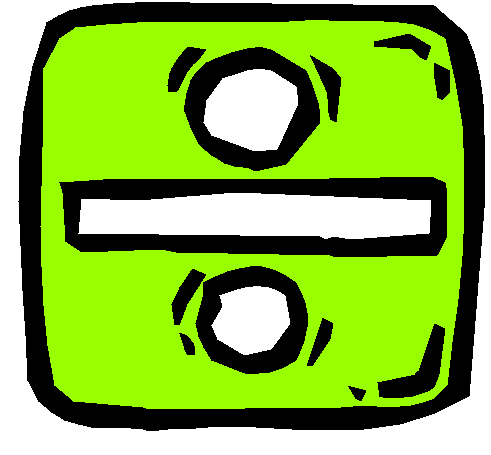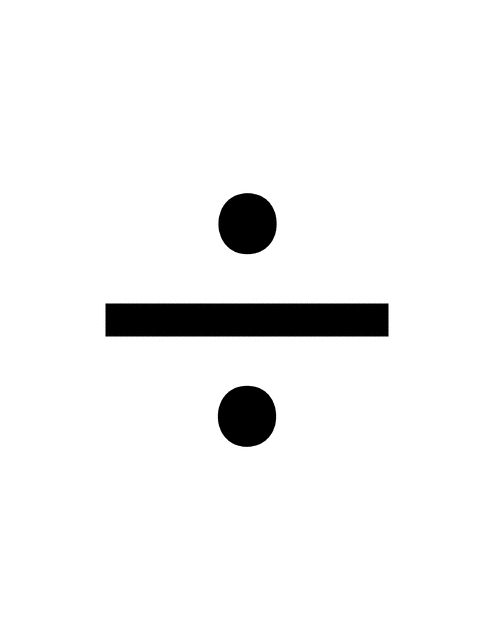Division Explosion!In Maths we are focusing on exploring the concept of DIVISION.
Division is the sharing or grouping a number into equal parts.  In a division equation this first number is the total being divided then there is the division symbol.  The next number is the number that will divide the total.  The answer is the result of the dividing.  It is called the quotient.

Our Division Unit is pushing students to think deeply and carefully about division situations.  It highlights the two types of division situations, quotative and partitive, or grouping and sharing.

In a quotative, or grouping division situation, the number in each group is known and the number of groups is unknown:  24 fourth graders are playing soccer.  The coaches want to make teams of 6.  How many teams can they make?  6 is the number in each group, and the unknown is how many groups will be made with 24 kids.
In  a partitive, or sharing division situation, the number of groups is known and the number in each group is unknown:  I have 24 cookies to share with 6 friends.  How many cookies can I give to each friend?  6 is the number of groups, and the unknown is how many cookies will be in each group.  (This is the strategy we are most familiar with 🙂 )
In the classroom, we are talking about whether a division situation is asking “How many groups?” or “How many in each group?”

We are practicing how to

1. divide a collection of objects into equal-sized groups.
2. identify the difference between dividing a set of objects into equal groups (THIS IS QUOTITION) and dividing the same set of objects into groups of (THIS IS CALLED PARTITION)

Can you work out which strategy matches these stories?

Story One:

I have made 20 cookie balls and need to share them equally onto 4 trays to bake them.  How many cookies will be on each tray?

Story Two:

I have 20 cookies and will pack them into bags of 4.  How many bags will I need?

Can you draw me a picture to show the difference between each story?

Can you make up some division stories for your classmates to solve?

Can you make up a partition and quotition story to match 243 ?

4 thoughts on “Division Explosion!”

1.luca on said:

Hi Miss M,
I know a different strategy for division. It is multiplying by the divisor until you get to the addend. Eg. 28/7 = 7 x 4, 28/7 = 4.
Division is awesome and I also like how at school, we count the number in each group. That was Partition.
I hope we learn some more division strategies.
See you at school,
From Luca.

2.amorse on said:

That is great thinking Luca. We are going to look at that strategy on Wednesday 🙂

3.Cooper on said:

I have been loving division! It has been the best this term.

Have you been liking division?

4.amorse on said:

Absolutely Cooper. Division has been a lot of fun to learn about. Maths calculations are awesome 🙂